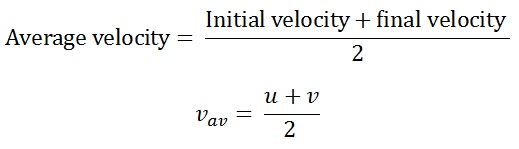Study Materials: CBSE Notes

Our CBSE Notes for Notes 8. Motion - Velocity | Class 9 Science - Toppers Study is the best material for English Medium students cbse board and other state boards students.

Notes ⇒ Class 9th ⇒ Science ⇒ 8. Motion

# Notes 8. Motion - Velocity | Class 9 Science - Toppers Study

Topper Study classes prepares CBSE Notes on practical base problems and comes out with the best result that helps the students and teachers as well as tutors and so many ecademic coaching classes that they need in practical life. Our CBSE Notes for Notes 8. Motion - Velocity | Class 9 Science - Toppers Study is the best material for English Medium students cbse board and other state boards students.

## Notes 8. Motion - Velocity | Class 9 Science - Toppers Study

CBSE board students who preparing for class 9 ncert solutions maths and Science solved exercise chapter 8. Motion available and this helps in upcoming exams 2023-2024.

### You can Find Science solution Class 9 Chapter 8. Motion

• All Chapter review quick revision notes for chapter 8. Motion Class 9
• NCERT Solutions And Textual questions Answers Class 9 Science
• Extra NCERT Book questions Answers Class 9 Science
• Importatnt key points with additional Assignment and questions bank solved.

Chapter 8 Science class 9

### Velocity class 9 Science Chapter 8. Motion

• Notes 8. Motion - Velocity | Class 9 Science - Toppers Study
• Class 9 Ncert Solutions
• Solution Chapter 8. Motion Class 9
• Solutions Class 9
• Chapter 8. Motion Velocity Class 9

## Notes 8. Motion - Velocity | Class 9 Science - Toppers Study

### 8. Motion

(Science-IX)

Speed with Direction:

Velocity:

The direction of motion along with its speed. The quantity that
specifies both these aspects is called velocity.

Defintion: Velocity is the speed of an object moving in a definite direction. It is called velocity.

S.I unit of velocity is m/s or m s-1.

Type of Velocity:

(i) Uniform velocity: When an object covers equal displacement in equal time of interval. It is called uniform velocity.

(ii) Non-uniform velocity: When a object covers unequal displacement in equal time of interval. It is called non-uniform velocity.

(iii) Average velocity: Average velocity is given by the arithmetic mean of
initial velocity and final velocity for a given period of time.Initial velocity : Initial velocity of an object means the velocity when the object starts displacing where time(t) may be zeto or t ≠ 0.

It is denrted by 'u'.

When object starts moving from rest then u = 0.

When an object is in motion then u ≠ 0. The value will be any integer.

Final velocity : Final velocity of an object is given by time t. It is the value of final position in velocity of the object.

• Initial and final velocity of an object can be zero.
• When an object starts from rest its initial velocity is zero.
• If it stops after applying break,its final velocity will be zero. As object is in rest.

Differences between speed and velocity;

Speed:

(i) It is a distance covered in unit time.

(ii) It is a scalar quantity.

(iii) Average speed of moving object can not be zero.

(iv) Speed of an object can never be zero.

Velocity:

(i) It is a displacement covered in unit time with direction.

(ii) It is a vector quantity.

(iii) Average velocity of moving object can be zero.

(iv) Velocity of an object can be positive, negative or zero.

When the object is moving in a straight line along positive direction and the distance and displacement of an two different objects are equal in same time then the value of speed and velocity will be equal.

Changing the velocity of an object:

(i) Change its magnitude.

(ii) To change its the direction.

(iii) To change its both magnitude and direction.

Locomotion:

Motion of animals is known as locomotion.

Important Study materials for classes 06, 07, 08,09,10, 11 and 12. Like CBSE Notes, Notes for Science, Notes for maths, Notes for Social Science, Notes for Accountancy, Notes for Economics, Notes for political Science, Noes for History, Notes For Bussiness Study, Physical Educations, Sample Papers, Test Papers, Mock Test Papers, Support Materials and Books.Mathematics Class - 11th

NCERT Maths book for CBSE Students.

books

## Study Materials List:

##### Notes ⇒ Class 9th ⇒ Science
1. Matter in Our Surroundings
2. Is Matter around us Pure
3. Atoms and Molecules
4. Structure of The Atom
5. The Fundamental Unit of Life
6. Tissues
7. Diversity in Living Organisms
8. Motion
9. Force and Laws of Motion
10. Gravitation
11. Work and Energy
12. Sound
13. Why Do We Fall ill
14. Natural Resources
15. Improvement in Food Resources

New Books